# GSEB Solutions Class 12 Maths Chapter 1 Relations and Functions Ex 1.3

Gujarat Board GSEB Textbook Solutions Class 12 Maths Chapter 1 Relations and Functions Ex 1.3 Textbook Questions and Answers.

## Gujarat Board Textbook Solutions Class 12 Maths Chapter 1 Relations and Functions Ex 1.3Question 1.
Let f : {1. 3 4} → {1,2, 5} and g: {1, 2, 5} → {1,3} be given by f = {(1, 2), (3, 5), (4,1)} and g = {(1, 3), (2, 3), (5,1)}. Write down
Solution:
f= {(1,2), (3,5), (4,1)}
g = {(I, 3), (2, 3), (5, 1)}
(f) = 2, g(2) = 3 ⇒ gof(1) = 3
f(3) = 5, g(5) = 1 ⇒ gof(3) = 1
f(4) = 1, g(1) = 3 ⇒ gof(4) = 3
⇒ gof = {(1,3), (3,1), (4, 3)].

Question 2.
Let f, g and h be functions from R to R. Show that
(i) (f + g)oh = foh + goh.
(ii) (f. g)oh = (foh) . (goh).
Solution:
f : R → R, g : R → R, h : R → R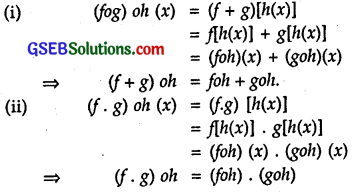Question 3.
Find gof and fog, if $$x^{\frac{1}{3}}$$
(i) f(x) = |x|, and g(x) = |5x – 2|.
(ii) f(x) = 8x³ and g(x) = $$x^{\frac{1}{3}}$$
Solution:
(i) f(x) = |x|, and g(x) = |5x – 2|

• gof(x) = g[f(x)]=g|x| = |5|x|-2|.
• fog(x) = f[g(x)] = f(|5x- 2|) =||5x-2|| = |5x – 2|.

(ii) f(x) = 8x³ and g(x) = $$x^{\frac{1}{3}}$$

• gof(x) = g[f(x)] = g(8x³) = (8x³)$$x^{\frac{1}{3}}$$ = 2x.
• fog(x) = f[(g(x)] = f($$x^{\frac{1}{3}}$$) = 8.($$x^{\frac{1}{3}}$$)³ = 8x.Question 4.
If f(x) = $$\frac { 4x+3 }{ (6x-4) }$$, x ≠ $$\frac { 2 }{ 3 }$$ show that fof(x) = x for all x ≠ $$\frac { 2 }{ 3 }$$ What is the inverse of f?
Solution:
f(x) = $$\frac { 4x+3 }{ (6x-4) }$$, x ≠ $$\frac { 2 }{ 3 }$$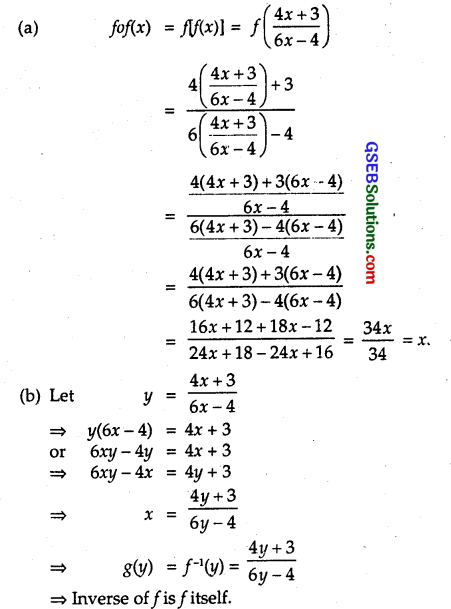Question 5.
State with reasons whether each of the following functions has a inverse or not:
Solution:
(i) f : {1, 2, 3, 4} → {10} with
f= {(1, 10), (2, 10), (3, 10), (4, 10)}.

(ii) g: {5, 6, 7, 8} → {1, 2, 3, 4} with
g = {(5, 4), (6, 3), (7, 4), (8, 2)}.

(iii) h : {2, 3, 4, 5} → {7, 9, 11, 13} with
h = {(2, 7), (3, 9), (4, 11), (5, 13)}.
Solution:
(i) f : {1, 2, 3, 4} → {10} with
f= {(1, 10), (2, 10), (3, 10), (4, 10)}.
f is not one-one since 1, 2, 3, 4 have same page 10.
⇒ f has no inverse.

(ii) g: {5, 6, 7, 8} → {1,2, 3,4} with
g = {(5, 4), (6, 3), (7, 4), (8, 2)}.
Here also 5 and 7 have the same image 4.
∴ g is not one-one.
Therefore, g is not invertible, i.e., It has no inverse.

(iii) h : {2,3,4,5} → {7,9,11,13} with
h = {(2, 7), (3, 9), (4, 11), (5, 13)}.
Hence, f(x1) = f(x2) ⇒ x1 = x2.
i. e., each element of X = {2,3,4,5} has a unique image in Y = {7,9,11,13}.
Similarly, each member of Y has a unique pre-image in X.
⇒ f is one-one and onto.
∴ f is invertible, i.e., It has an inverse.Question 6.
Show that f: [- 1, 1] → R, given by f(x) = $$\frac { x }{ x+2 }$$, x ≠ – 2 is one-one. Find the inverse of the function f : [- 1, 1} → Range f.
Solution: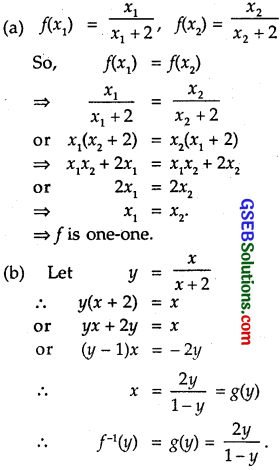Question 7.
Consider f : R → R given by f(x) = 4x + 3. Show that f is invertible. Find the inverse of f.
Solution:
f : R → R given by f(x) = 4x + 3.
f(x1) = 4x1 + 3, f(x2) = 4x2 + 3
If f(x1) = f(x2),
then 4x1 + 3 = 4x2 + 3
or 4x1 = 4x2
⇒ x1 = x2.
⇒ f is one-one.
Aslo, let y = 4x + 3
or 4x = y – 3
∴ x = $$\frac { y-3 }{ 4 }$$.
For each value of y e R and belonging to codomain of y, there is a pre-image in its domain.
∴f is onto.
i. e., f is one-one and onto.
∴ f is invertible.
So, f-1(y) = g(y) = $$\frac { y-3 }{ 4 }$$

Question 8.
Consider f : R+ → [4, ∝) given by f(x) = x² + 4. Show that f is invertible with the inverse of f-1 of f given by f-1(y) = $$\sqrt{y-4}$$, where R+ is the set of all non-negative real numbers.
Solution:
f(x1) = x²1 + 4 and f(x2) = x²2 + 4
So, f(x1) = f(x2) ⇒ x²1 + 4 = x²2 + 4
or x²1 = x²2 ⇒ x1 = x2
As x ∈ R, ∴ x > 0,
1 = x²2 ⇒ x1 = x2
⇒ f is one-one.
Let y = x² + 4 or x² = y – 4 or x = ± $$\sqrt{y-4}$$.
x being > 0, -vc sign not to be taken.
∴ = $$\sqrt{y-4}$$
∴ f-1(y) = g(y) = $$\sqrt{y-4}$$, y ≥ 4.
For every y ≥ 4, g(y) has real positive value.
∴ The inverse of f is f-1(y) = $$\sqrt{y-4}$$.

Question 9.
Consider f : → R+ [- 5, ∝) given by f(x) = 9x² + 6x – 5. Show that f is invertible with f-1(y) = $$\left(\frac{\sqrt{y+6}-1}{3}\right)$$
Solution:
Let y be an arbitrary element in the range of f.
Let y = 9x² + 6x – 5
= 9x² + 6x + 1 – 6
= (3x +1)² – 6
⇒ y + 6 = (3x + 1)²
or 3x + 1 = $$\sqrt{y+6}$$
∴ x = $$\left(\frac{\sqrt{y+6}-1}{3}\right)$$ = g(y).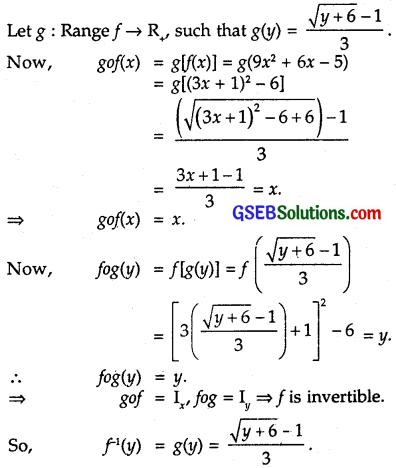Question 10.
Let f : X → Y be an invertible function. Show that f has a unique inverse.
Solution:
If f is invertible, then gof(x) = Ix and fog(y) = Iy.
⇒ f is one-one and onto.
Let there be two inverses g1 and g2
∴ fog1 (y)= Iy, f0g2(y) = Iy
Iy being unique for a given function f, we get
g1(y) = g2(y) [ ∵ f is one-one and onto.]
∴ f has a unique inverse.Question 11.
Consider f : {1, 2, 3} → {a, b, c] given by f(1) = a, f(2) – b, f(3) = c. Find f-1 and show that (f-1)-1 = f.
Solution:
f : {1, 2, 3} → {a, b, c}
so that f(1) = a, f(2) = b, f(3) = c.
Now, let X = {1, 2, 3}, Y = {a, b, c).
∴ f : X → Y
∴ f-1 : Y → X such that f-1(a) = 1, f-1(b) = 2, f-1(c) = 3.
Inverse of this function may be written as
(f-1)-1 : X → Y such that (f-1)-1 = a, (f-1)-1(2) = b and (f-1)-1(3) = c.
We also have:
f: X Y, such that f(1) = a, f(2) = b, f(3) = c.
⇒ (f-1)-1 = f

Question 12.
Let f : X → Y be an invertible function. Show that the inverse of f-1 is f, i.e., (f-1)-1 = f.
Solution:
f : X → Y is an invertible function.
∴ f is one-one and onto ⇒g : Y → X, where g is also one-one and onto such that
gof(x) = Ix and fog(y) = Iy ⇒ g = f-1.
Now, f-1o(f-1)-1 = I
and fo[f-1o(f-1)-1] = foI
or (fof-1)o(f-1)-1 = f
Io(f-1)-1 = f
⇒ (f-1)-1 = f

Question 13.
If f : R → R be given by f(x) = (3-x³)$$\left(3-x^{3}\right)^{\frac{1}{3}}$$, when fof(x) is
(A) $$x^{\frac{1}{3}}$$
(B) x³
(C) x
(D) 3-x³.
Solution: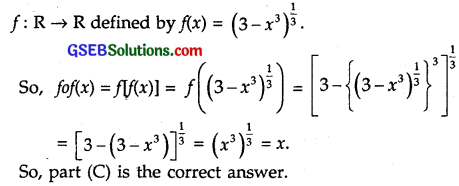Question 14.
Let f : R – (- $$\frac { 4 }{ 3 }$$) → R be a function, defined as f(x) = $$\frac { 4x }{ 3x+4 }$$, x ≠ – $$\frac { 4 }{ 5 }$$.
The inverse off is map g: Range f → R – is given by $$\frac { 4 }{ 3 }$$ is given by
(A) g(y) = $$\frac { 3y }{ 3-4y }$$
(B) g(y) = $$\frac { 4y }{ 4-3y }$$
(C) g(y) = $$\frac { 4y }{ 3-4y }$$
(D) g(y) = $$\frac { 3y }{ 4-3y }$$
Solution:
f : R – {$$\frac { -4 }{ 3 }$$} → R is a function defined by f(x) = $$\frac { 4x }{ 3x+4 }$$
Let y = $$\frac { 4x }{ 3x+4 }$$.
⇒ 3xy + 4y = 4x
or x(4 – 3y) = 4y
∴ x = $$\frac { 4y }{ 4-3y }$$
∴ f-1(4y) = g(y) = $$\frac { 4y }{ 4-3y }$$
So, part (B) is the correct answer.